# Hidden Markov Models: an Overview

By Field Cady, Aug 31, 2021

There are many tools available for analyzing sequential data. One of the most simple, flexible and time-tested is Hidden Markov Models (HMMs). They were originally developed for signal processing, and are now ubiquitous in bioinformatics.

In the data science community there is a tendency to favor machine learning options like LSTMs. While those tools are quite powerful, they are also notoriously complicated and difficult to understand. As a result you often see data scientists struggling to use a complicated approach when a simple one would work better.

This article aims to fix that, bringing technologists up to speed on the concepts and applications of the often-overlooked HMM. At Zeitworks we rely heavily on state-of-the-art machine learning technologies, but as experienced data scientists we also know that sometimes an HMM is the tool for the job.

# Motivating Real-World Examples

Here are some problems for which I have used HMMs:

• At Zeitworks we study human workflows using computer event logs. Part of that is taking a user’s raw event log (keystrokes, URLs visited, etc.) and determining what they were doing at each time (background research, document review, etc.). Sometimes you can guess from one event in isolation, but usually you also need to look at the events before and after to have confidence.

• You have a photograph of a page from a novel, and you want to digitize the text. The photograph is already segmented into images of words. Most of them can be decoded with OCR, but some words are ambiguous because of dirt on the original page (is that “quick” or “quack”?). You want to use the surrounding words to pick the best option.

• You have a sequence of brain scans, taken every second while a person was playing a video game. During the game their avatar moves in and out of buildings, and your goal is to guess whether it is inside or outside using only the brain scans. Any given scan is very ambiguous, but the avatar usually stays inside/outside for many seconds at a time. You expect large spans of time where the avatar is in(out)side, and where the brain scans look slightly more in(out)side on average.

It’s possible to set up a custom solution to each of these problems, but it’s an extremely large undertaking that runs the risk of being frail or hacky. Oftentimes, especially if the work is partly exploratory, you want something lighter weight. HMMs provide a framework that is general enough to cover all these situations, flexible enough to bake in a lot of domain knowledge, and simple enough to make sense of.

# Mathematical Model

Let’s transition from the business realities to mathematical idealizations. The above business problems have several things in common:

• There is a discrete underlying real-world “state” that changes over time. In examples (1) and (3) the state changes only rarely. In example (2) the state is the word on the page, and it changes almost every time step. Let’s call this real-world state S.

• At each timestep you have an “observation” that can be used to guess at the state with some level of confidence (for the OCR example confidence is usually high, but for brain scans it is low). Let’s call the observation O. Note that while the state S is discrete, O can be discrete or continuous.

• The idea is to use the observation O at time T to guess at the state S, but then refine our guess using the observations before and after T.

The overall picture looks like this, where the states follow one after another, and each one gives rise to an observation: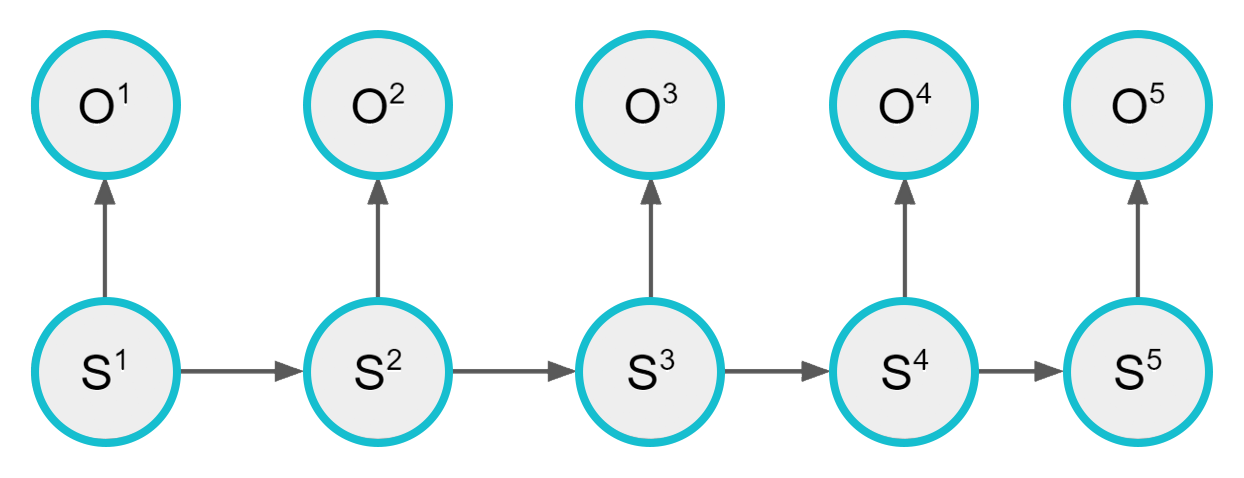The dependency structure between states and observations in an HMM

For concreteness keep the following picture in your head. The states S are dice that can be rolled, and the observations O are which side comes up. For simplicity say there are two dice, where one is fair and the other is loaded with a 50% chance of 6. After rolling the fair dice there is a 10% chance I switch to the other dice, and after the loaded dice there is a 20% chance I switch. I make many rolls — without ever telling you which die I’m using — and your job is to guess when the dice was fair or loaded. The final piece of information you need is how likely I am to use the fair versus the loaded dice for the first roll; let’s call that one 50/50. The situation can be summarized in three tables: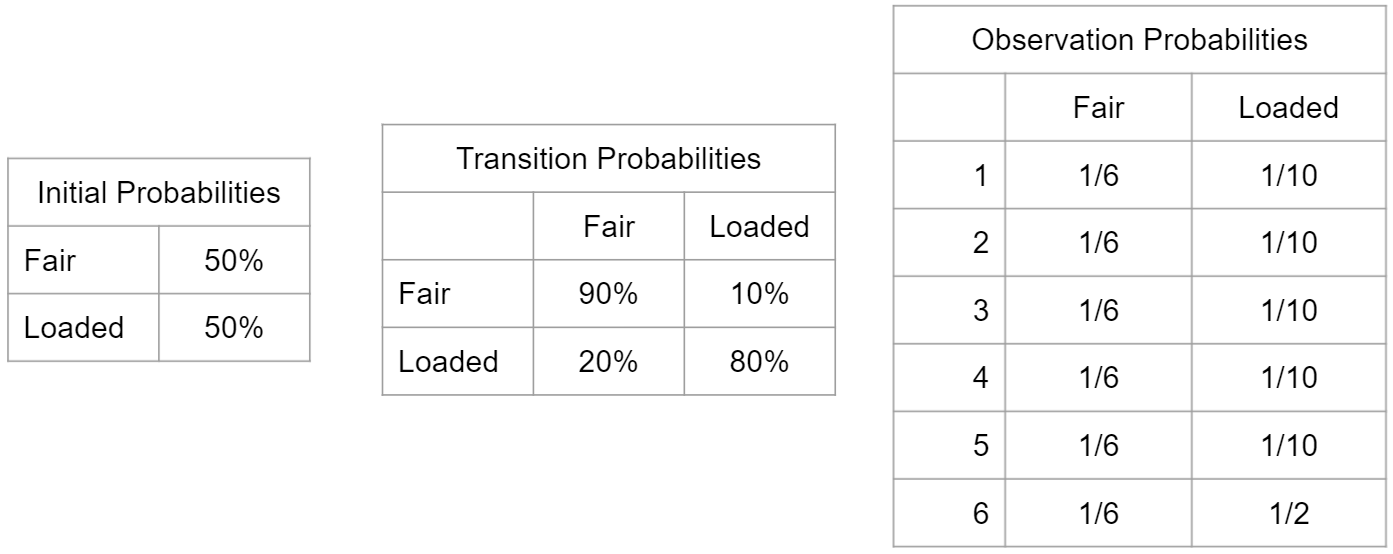The mathematical description of a simple HMM with the dice, one loaded and one fair.

To express this in mathematical terminology we need:

• The initial probabilities of each state, P[S⁰=s]. This vector is often called π.

• The matrix of transition probabilities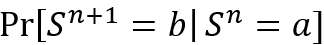which for simplicity I will call Pr[a → b].

• The probability distribution for the observation given the state, Pr[O=o|S=s]. For the dice O is discrete, and the probability distribution gets put in a table. But mathematically O can be anything; for the algorithms we will just need a function that takes in the state (an integer from 1 to k) and the observation (which can be anything) and returns a non-negative number.

I should note that if we forget about observations, the states S constitute a simpler model called a “Markov chain”. Pretty much everything in stochastic analysis is either a Markov chain or a variant of one; HMMs are a variant where the states of the chain are hidden from our knowledge (hence the name) and must be guessed via imperfect observations.

# Limitations and Optimizations of HMMs

I would like to discuss some of the idealizations in the HMM model that are rarely 100% true, as well as what you can do about it. You can skip this section if you just want to get to how to use HMMs :)

The biggest idealization is the so-called “Markov assumption”, that the next state is dependent only on the current state. I like to compare it to a frog with amnesia who is hopping between lily pads; he has no idea how long he has been on the current pad or where he was before, so he chooses the next pad (or not to hop at all) based only on what he sees from his current location. You can partly work around this by having an nth-order Markov chain, where your “state” is actually a sliding window that covers the last n states including the current one. You see this a lot in using HMMs for natural language. It becomes computationally dicey though because you now have k^n different “states” to keep track of.

The next assumption in HMMs is that the states are truly discrete. Usually a human decided what the states are, and the reality on the ground might not fit cleanly into just one of them. HMMs have no notion of hybrid states, but they do support uncertainty about the state; when the model indicates that two states are comparably likely it could be that the signal is low, but it could also be that the world is not fitting into our boxes.

Finally there is the all-important Pr[O|S], the probability of your observation given the underlying state. In our toy dice example it is just a look-up table. In the real-world examples I cited though, crafting Pr[O|S] was a project in its own right, involving modeling assumptions and fitting to historical data. An HMM puts no constraints on how complicated Pr[O|S] is, but it does assume that O depends only on the current S, not on any previous S or O. That assumption is not true of course- observations are often correlated because of real-world dynamics that aren’t captured in S- but in practice it is rarely problematic.

# Computing the States: the Viterbi Algorithm

Now let’s put this model into practice! There are a lot of things you can do with an HMM, but 90% of the time in business it is this: given a sequence of N observations, guess what the states were. For this we use the famed Viterbi algorithm, which computes the optimal sequence of underlying states given our observations.

When I say “optimal” I mean “most probable” — the probability of 1) getting that sequence of states from the underlying Markov chain, and then 2) that sequence generating the known observations.

To do this consider a related subproblem: finding the optimal sequence up to timestep i that ends in a particular state s. Let’s define σ(i, s) to be the probability of that sequence. Think about the dice example: the key insight is that the best sequence up to i+1 that ends is Fair is either {the best up to i that ends in Fair}+{Fair}, or {the best up to i that ends in Loaded}+{Fair}. If we know the best sequences up to time i, then i+1 is easy! In math this tells us that: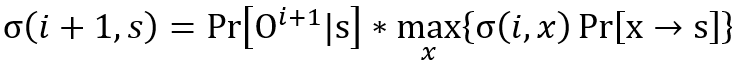We can arrange all the σ(i, s) in a table like this: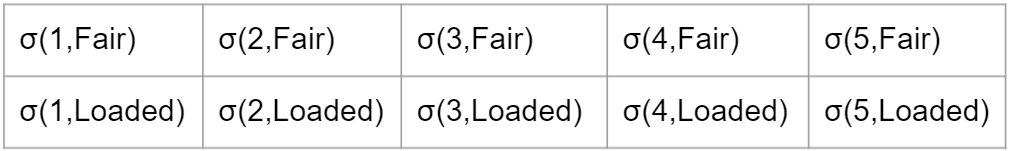Once this table is populated we are almost done with the Viterbi algorithm! We can find the last state in the optimal sequence by seeing which s maximizes σ(N, s). Then we work backward — in computing σ(N, s) which x did we use for σ(N-1, x)? You can visualize the process by drawing an arrow from σ(i, s) to σ(i-1, x) like this: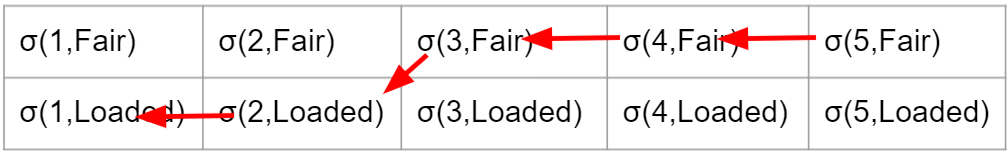In this way we can reconstruct the entire optimal sequence. If we want to express this is pseudo-code we can write: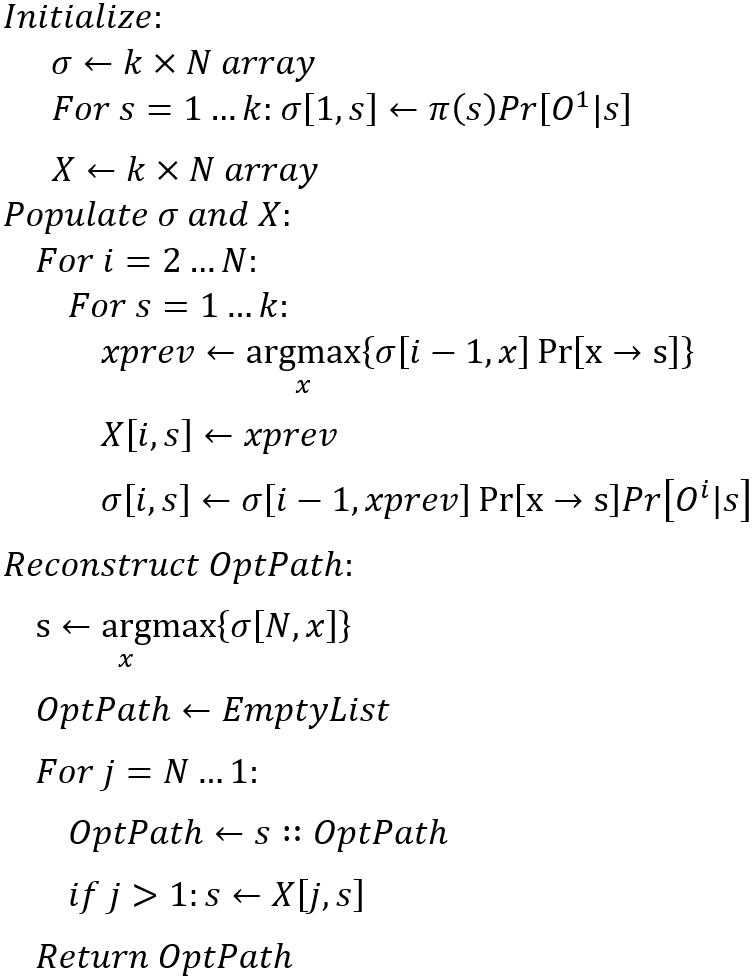There is only one small implementation detail that I must note. The algorithm as stated will run into numerical problems because the probabilities become so small. This is easily solved by operating on the logs of probabilities, and computing a table of Log[σ(i, s)] instead of σ(i, s). Maximizing Log[σ(i, s)] is equivalent to maximizing σ(i, s).

# Parting Words

When I tell people in the tech industry about what we do at Zeitworks the typical response I get is “oo, you could use an LSTM for that!”. And while those are certainly in vogue these days, there are many tools for analyzing sequential data and they each have their strengths.

LSTMs — and other deep learning models- shine in situations where the real-world patterns are not intelligible. These are “data-driven” tools; with enough data to train on, and enough knobs to turn, they can learn to ape complicated patterns that no human ever wrote down. In exchange they are hard to interpret, difficult to generalize if a problem changes, and reliant on massive amounts of data.

HMMs are the opposite. They are “model-driven”: a human-friendly narrative about the world that has been distilled down to equations. The parameters in those equations get fitted to data, but they are meaningful numbers with a real-world interpretation rather than a piece of numerical voodoo. Model-driven tools are less powerful than data-driven ones, but in exchange they are cheap to train, easy to understand, and you can bake expert knowledge into them.

Data-driven and model-driven tools are complementary, not antagonistic. They are ideal for different problems, and at Zeitworks we incorporate them both. But doing this hinges on the ability to think critically about all of the tools in your arsenal, understanding their strengths so that you can match them to the right tasks.

My goal in this article has been to give you that understanding with HMMs. They are powerful models — though often underappreciated in the data science community- and they are often the best tool for the job.